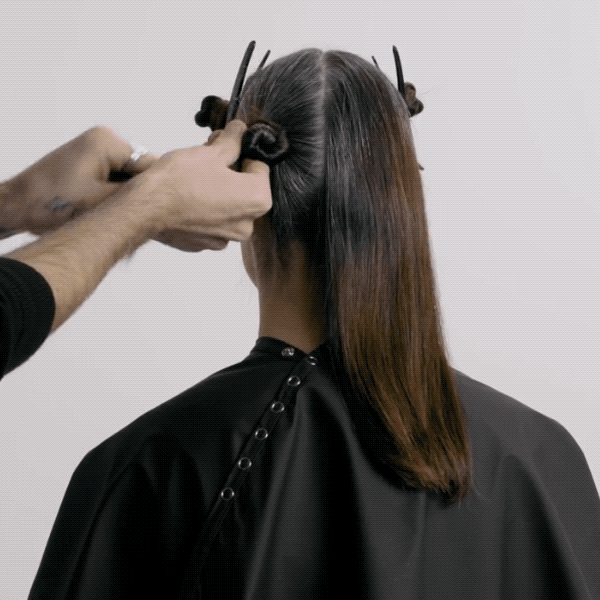Handpainted Brunette + Gray Retouch
November 9, 2017

# Handpainted Brunette + Gray Retouch

VIEW ALL PHOTOS VIEW SLIDESHOW
GET THE HOW-TO• Formula A

• Formula B

• Formula C# Handpainted Brunette + Gray Retouch

Keep your gray coverage clients from getting stuck in a rut by adding a subtle handpainting technique to her regular retouch. This look by TIGI® Global Technical Academy Director Warren Boodaghians adds dimension and amps up shine with 100 percent gray coverage. Check it out!

Colorist: Warren Boodaghians
Manufacturer: TIGI, @tigiprofessionals

• Formula A

• Formula B

• Formula C

## HOW-TO STEPS•• Formula A

• Formula B

• Formula C

1

Natural Level: 5

Percentage of non-pigmented hair: 50-70%

•• Formula A

• Formula B

• Formula C

2

Create a profile parting, then a radial parting, for a total of four sections. Secure all sections with TIGI® Pro Sectioning Clips.

•• Formula A

• Formula B

• Formula C

3

Begin at the nape and apply Formula A in horizontal partings on the new growth and midlengths. Continue until the back is complete.

••• Formula A

• Formula B

• Formula C

4

Move onto the side sections and take slight diagonal partings. At the first parting, apply Formula A to the new growth and midlengths. On the next two partings, apply Formula A to the new growth and midlengths, then freehand-paint Formula B.

•• Formula A

• Formula B

• Formula C

5

Continue working, alternating the technique in a 1:1 ratio until the section is complete. Repeat on the opposite side.

•• Formula A

• Formula B

• Formula C

6

Process for 30 minutes, rinse and remove, then tone the prelightened areas with Formula C.

•• Formula A

• Formula B